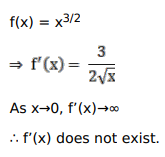# Solve this following

Question:

Mark $(\sqrt{)}$ against the correct answer in the following:

Let $f(\mathrm{x})=\mathrm{x}^{3 / 2}$. Then, $f^{\prime}(0)=$ ?

A. $\frac{3}{2}$

B. $\frac{1}{2}$

C. does not exist

D. none of these

Solution: